###### 文件的操作

``````int main()
{
int a = 10000;
FILE* pf = fopen("test.txt", "wb");
fwrite(&a, 4, 1, pf);
fclose(pf);
pf = NULL;
return 0;
}``````

ANSIC标准采用“缓冲文件系统”处理的数据文件，所谓缓冲文件系统是指系统自动地在内存中为程序中每一个正在使用的文件开辟一块“文件缓冲区”。 从内存向磁盘输出数据会先送到内存中的缓冲区，装满缓冲区后才一起送到磁盘上。如果从磁盘向计算机读入数据，则从磁盘文件中读取数据输入到内存缓冲区（充满缓冲区），然后再从缓冲区逐个地将数据送到程序数据区（程序变量等)。 缓冲区的大小根据C编译系统决定的。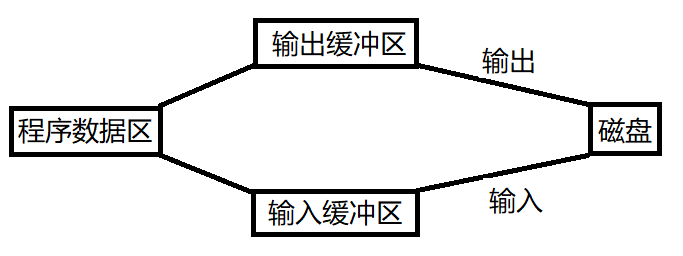``````//绝对路径：
FILE* pf = fopen("C:\\2020\\code\\test.txt", "r");
//相对路径
FILE* pf = fopen("test.txt", "r");``````::: tip 当要打开的文件和代码文件在同一个路径下时，可以写成相对路径的形式。 绝对路径写法时，“\”会被识别成转义字符，需要在“\”后再加一个“\”，对每一个“\”都进行转义，就不会再识别成转义字符了。 :::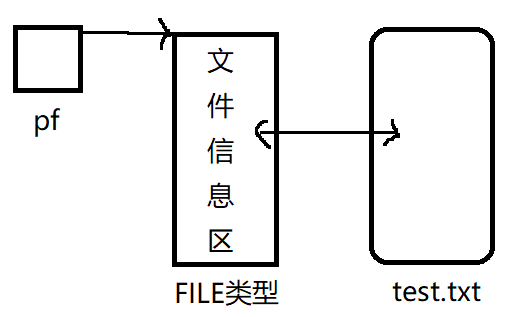创建文件test.txt时，系统会自动创建填充test.txt的文件信息区，并将文件信息区的起始地址返回给pf。 ::: warning 当打开文件失败时，FILE结构体不会创建（不创建文件信息区），fopen返回空指针，pf中填充NULL。 ::: 读文件完毕后需要关闭文件释放空间，fclose(pf)是值传递，不改变pf的值，需要手动pf=NULL，类似于free释放空间。 读文件的全过程：

``````#include <errno.h>
#include <string.h>
int main()
{
FILE* pf = fopen("../test.txt", "r");
if (pf == NULL)
{
printf("%s\n", strerror(errno));
return 0;
}
//文件打开成功
//读文件
//关闭文件
fclose(pf);
pf = NULL;
return 0;
}    ``````

###### 文件的顺序读写

``````int main()
{
int ch = fgetc(stdin);
fputc(ch, stdout);
return 0;
}``````
``````h
h``````

``````#include <errno.h>
#include <string.h>
int main()
{
FILE* p = fopen("test.txt", "r");
if (p == NULL)
{
printf("%s\n", strerror(errno));
return 0;
}
//写文件
//fputc('H', p);
//fputc('i', p);
//读文件
int ch = fgetc(p);
printf("%c ", ch);
ch = fgetc(p);
printf("%c ", ch);
//关闭文件
fclose(p);
p = NULL;
return 0;
}``````
``H i``

::: tip 如上述代码，fgetc在读取时，每次读取一个字符，连续读写时可以自动向下跳。 :::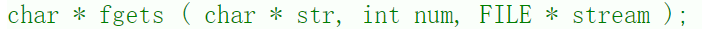stream：流，文件。 str：数据存储位置。从文件读取的信息放入str指向的字符串中。 n：最多读取n个字符。

``````#include <string.h>
#include <errno.h>
int main()
{
FILE* ph = fopen("test.txt", "r");
if (ph == NULL)
{
printf("%s\n", strerror(errno));
return 0;
}
char pph = { 0 };
//char* fgets(char* str, int num, FILE * stream);
fgets(pph,1024,ph);
printf("%s", pph);
fgets(pph, 1024, ph);
printf("%s", pph);
fclose(ph);
ph = NULL;
return 0;
}``````
``````Hi
Cat``````

puts：写一行到标准输出（屏幕）。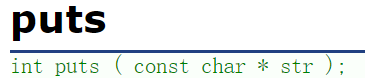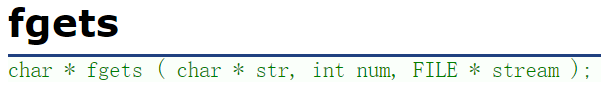``````#include <string.h>
#include <errno.h>
int main()
{
FILE* ph = fopen("test.txt", "r");
if (ph == NULL)
{
printf("%s\n", strerror(errno));
return 0;
}
char pph = { 0 };
//char* fgets(char* str, int num, FILE * stream);
fgets(pph,1024,ph);
puts(pph);
fgets(pph, 1024, ph);
puts(pph);
fclose(ph);
ph = NULL;
return 0;
}``````
``````Hi

Cat
``````

puts在打印完一行内容后会自动换行。

fputs：写一行内容到流中。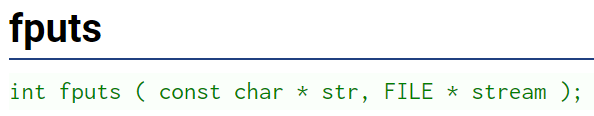``````#include <string.h>
#include <errno.h>
int main()
{
FILE* ph = fopen("test.txt", "w");
if (ph == NULL)
{
printf("%s\n", strerror(errno));
return 0;
}
fputs("Hello", ph);
fputs("World", ph);
fclose(ph);
ph = NULL;
return 0;
}``````fputs在写入时，如果需要换行，需要手动加上“\n”。

::: tip 文本行输入输出函数（fgets、fputs）一次操作一行内容。 :::

fgets和fputs适用于任何输入输出流：

``````int main()
{
char buf = { 0 };  //从键盘读取一行文本信息
//fgets(buf,100,stdin);  //从标准输入流读取
//fputs(buf, stdout);  //输出到标准输出流
gets(buf);
puts(buf);
return 0;
}``````

gets、puts和fgets、fputs的区别是前者是默认是从标准输入读，输出到标准输出上，期间没有发生过传参。

``````struct S
{
int n;
float score;
char arr;
};
int main()
{
struct S s = { 20,3.14f,"Hello" };  //3.14f表示是浮点型
FILE* pf = fopen("test.txt", "w");
if (pf == NULL)
{
return 0;
}
//格式化形式写入文件
fprintf(pf, "%d %f %s", s.n, s.score, s.arr);
//将结构体s中的信息写入到文件test.txt中
return 0;
}``````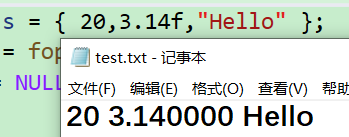上述代码第16行写入数据时可以调整写入顺序，只要格式和内容对应即可。文件中的数据顺序和写入顺序一致。 ::: tip 对比scanf和fscanf：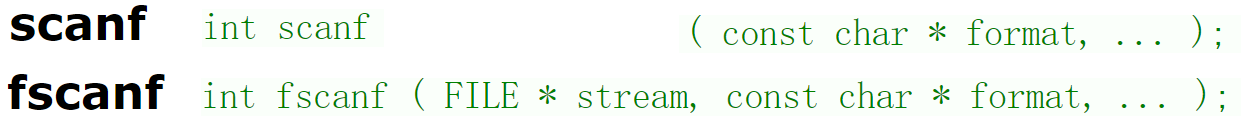:::

``````struct S
{
int n;
float score;
char arr;
};
int main()
{
struct S s = { 0 };
FILE* pf = fopen("test.txt", "r");
if (pf == NULL)
{
return 0;
}
//格式化输入数据（从文件中读取的数据放入结构体s）
fscanf(pf, "%d %f %s", &(s.n), &(s.score), s.arr);
printf("%d %f %s\n", s.n, s.score, s.arr);
return 0;
}``````
``20 3.140000 Hello``

fscanf和fprintf适用于任何输入输出流：

``````struct S
{
int n;
float score;
char arr;
};
int main()
{
struct S s = { 0 };
fscanf(stdin, "%d %f %s", &(s.n), &(s.score), s.arr);  //标准输入
fprintf(stdout,"%d %f %s\n", s.n, s.score, s.arr);  //标准输出
return 0;
}``````
``````1024 3.14 hi
1024 3.140000 hi``````

::: tip 上述代码中第10行，需要输入的数据内容的地址，s.arr本身就是数组s.arr[]的地址。 上述代码中第11行，如果需要控制打印的浮点数位数，比如精确控制打印出3.14，可以在%f之间加上“.2”，即%.2f可以打印两位浮点数。 :::

::: tip 一组函数的对比： scanf/printf：针对标准输入流/标准输出流的格式化输入/输出语句。 fscanf/fprintf：针对所有输入流/所有输出流的格式化输入/输出语句。 所有输入流/所有输出流包含标准输入/输出流和文件输入/输出流。

::: sscanf和sprintf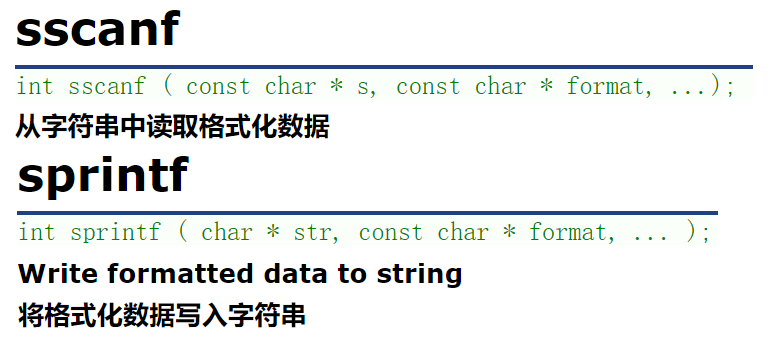``````struct S
{
int n;
float score;
char arr;
};
int main()
{
struct S s = { 20,3.14f,"Hello" };
char buf;
sprintf(buf, "%d %f %s", s.n, s.score, s.arr);
printf("%s", buf);
return 0;
}``````
``20 3.140000 Hello``

``````struct S
{
int n;
float score;
char arr;
};
int main()
{
struct S s = { 20,3.14f,"Hello" };
struct S tmp = { 0 };
char buf;
//把格式化的数据转换成字符串存储到buf中
sprintf(buf, "%d %f %s", s.n, s.score, s.arr);
//从buf中读取格式化的数据到tmp中
sscanf(buf, "%d %f %s", &(tmp.n), &(tmp.score), tmp.arr);
printf("%d %f %s\n", tmp.n, tmp.score, tmp.arr);
return 0;
}``````
``20 3.140000 Hello``

``````struct S
{
char name;
int age;
double score;
};
int main()
{
struct S s = { "张三",26,66.8 };
FILE* pf = fopen( "test.txt","wb" );
if (pf == NULL)
{
return 0;
}
//二进制形式写入
fwrite(&s, sizeof(struct S), 1,pf);
fclose(pf);
pf = NULL;
return 0;
}``````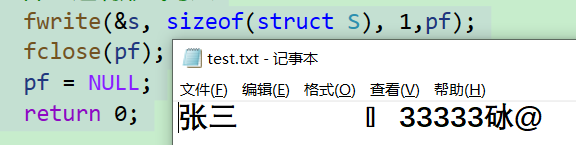上述代码输出结果乱码的原因是以二进制形式输出的。

``````struct S
{
char name;
int age;
double score;
};
int main()
{
//二进制形式读取
struct S tmp = { 0 };
FILE* pf = fopen("test.txt", "rb");
if (pf == NULL)
{
return 0;
}
printf("%s %d %lf\n", tmp.name, tmp.age, tmp.score);
fclose(pf);
pf = NULL;
return 0;
}
``````
``张三 26 66.800000``

::: tip 上述代码中一次可以写入读取一个数据，如果需要读取多个数据，上述代码中的“s”、“tmp”必须有能力存储多个元素，即可以是一个数组，同时后面的参数count也要改为需要读取的元素数量。 :::

fread的返回值：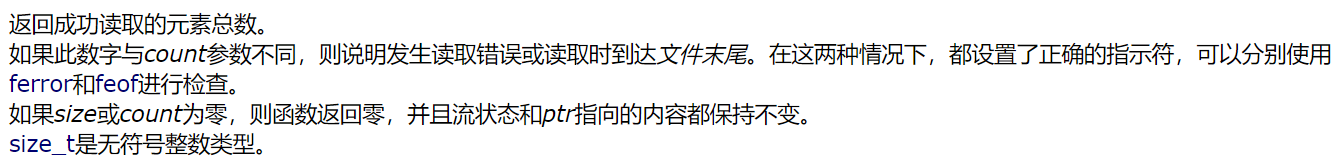::: warning 上图的意思是：假设参数count设置为10，如果返回5，则本次只读到5个元素。假设文件中有25个元素，第一次第二次都读到了10个元素，第三次读到了剩下的5个元素，即函数fread返回的值是真实读到的元素个数。 如果fread的返回值小于count参数的值，说明文件中的元素都被读完。 :::

###### 文件的随机读写

fseek 函数fseek的功能是根据文件指针的位置和偏移量来定位文件指针。（移动一个文件指针到指定位置）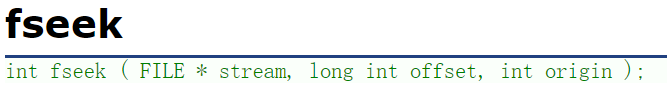stream：要调整位置的文件指针。 offset：偏移量，单位字节。 偏移量为正则向后偏移，偏移量为负则向前偏移。 origin：文件指针的当前位置。 起始位置origin的三个选项：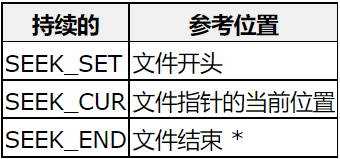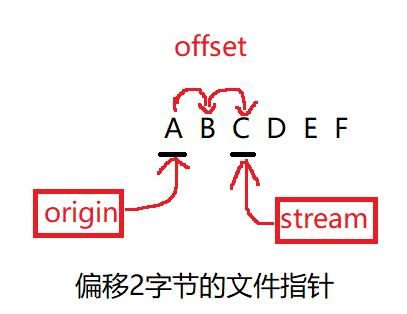如果没有指定偏移，默认的文件指针位置是起始位置。

``````int main()
{
FILE* pf = fopen("test.txt", "r");
if (pf == NULL)
{
return 0;
}
//定位文件指针
fseek(pf, 2, SEEK_SET);
//读取文件
int ch = fgetc(pf);
printf("%c\n", ch);
fclose(pf);
pf = NULL;
return 0;
}``````

ftell 函数ftell的功能是返回文件指针相对于起始位置的偏移量。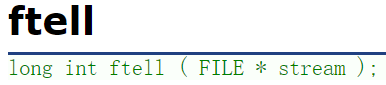``````int main()
{
FILE* pf = fopen("test.txt", "r");
if (pf == NULL)
{
return 0;
}
//定位文件指针
fseek(pf, -2, SEEK_END);
//输出文件指针偏移量
int pos = ftell(pf);
printf("%d\n", pos);
fclose(pf);
pf = NULL;
return 0;
}``````
``4``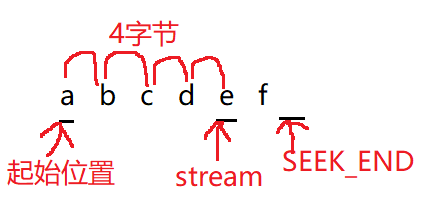``````int main()
{
FILE* pf = fopen("test.txt", "r");
if (pf == NULL)
{
return 0;
}
fgetc(pf);
int pos = ftell(pf);
printf("%d\n", pos);
fclose(pf);
fclose(pf);
pf = NULL;
return 0;
}``````

rewind 函数rewind的功能是让文件指针回到文件的起始位置。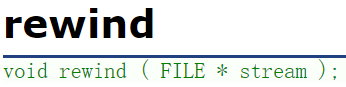``````int main()
{
FILE* pf = fopen("test.txt", "r");
if (pf == NULL)
{
return 0;
}
printf("%c\n", fgetc(pf));
printf("%c\n", fgetc(pf));
rewind(pf);
printf("%c\n", fgetc(pf));
fclose(pf);
pf = NULL;
return 0;
}``````
``````a
b
a``````

###### 文件结束判定

feof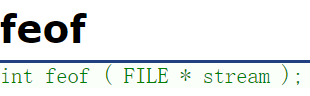::: warning feof不是EOF。

``````int main()
{
FILE* pf = fopen("test.txt", "r");
if (pf == NULL)
{
return 0;
}
int ch = fgetc(pf);
printf("%d\n", ch);
return 0;
}``````
``-1``

::: tip 涉及到perror perror的功能类似于strerror，但是更加简便。如下打开一个不存在的文件：

``````int main()
{
FILE* ph = fopen("test1.txt", "r");
if (ph == NULL)
{
perror("hehe");
return 0;
}
fclose(ph);
ph = NULL;
return 0;
}``````
``hehe: No such file or directory``

feof使用场景：

``````int main()
{
FILE* ph = fopen("test.txt", "r");
if (ph == NULL)
{
perror("hehe");
return 0;
}
//读取文件
int ch = 0;
while ((ch = fgetc(ph) != EOF))
{
putchar(ch);
}
if (ferror(ph))
{
printf("error\n");
}
else if (feof(ph))
{
printf("end of file\n");
}
fclose(ph);
ph = NULL;
return 0;
}``````Suzhou
Lv1

17

11

0